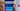# Calculate Max Profit - Buy and Sell Stocks Multiple Times - Leet Code Solution

August 27, 2020## Problem Statement

Say you have an array prices for which the ith element is the price of a given stock on day i.

Design an algorithm to find the maximum profit. You may complete as many transactions as you like (i.e., buy one and sell one share of the stock multiple times).

Examples

``````Input: [7,1,5,3,6,4]
Output: 7
Explanation:
- buy on day-2, sell on day-3. Profit (5-1) = 4
- buy on day-4, sell on day-5. Profit (6-3) = 3``````

## Solution (Simple)

Lets try a brute force algorithm. Since we know the constraint that we need to buy the stock before selling it.

• We can traverse the array.
• For every price, we can find if there is any following high price.
• If high price exists. Calculate profit, and run same algorithm on remaining array.

### Code

``````private int maxProfit_simpleRecursive(int[] prices, int startIndex) {
if (startIndex >= prices.length) {
return 0;
}

int maxProfit = 0;
for (int i=startIndex; i<prices.length; i++) {
int max = 0;
for (int j=i+1; j<prices.length; j++) {
//when can we sell
if (prices[j] > prices[i]) {
int profit = (prices[j] - prices[i]) + this.maxProfit_simpleRecursive(prices, j+1);
if (max < profit) {
max = profit;
}
}
}

if (maxProfit < max) {
maxProfit = max;
}
}

return maxProfit;
}

public int maxProfit_simple(int[] prices) {
return this.maxProfit_simpleRecursive(prices, 0);
}``````

Complexity
Above code runs in `O(n^n)`.

## A Better Solution

If we try to draw the input in some sort of graph or line chart. We can see some pattern.If you look closely, we can keep track of low points, and following high point. And take a sum of such points. We can get the maximum profit.

In the above image, we can take following calculation:

``````Low-point=1, High-point=5, Profit=4
Low-point=3, High-point=6, Profit=3

Total Profit = 7``````

### Code

``````public int maxProfit_better(int[] prices) {
if (prices == null || prices.length == 0) {
return 0;
}
int max = 0;
int i=0;

int lowPrice = prices[i];
int highPrice = prices[i];
i++;

while (i < prices.length) {
//find low value
while (i < prices.length && prices[i-1] >= prices[i]) {
i++;
}
lowPrice = prices[i-1];

//find high value
while (i<prices.length && prices[i] >= prices[i-1]) {
i++;
}
highPrice = prices[i-1];

max += (highPrice - lowPrice);
}

return max;
}``````

### Complexity

The code runs in `O(n)` time as we are running loop n times only.

### Better and Simple Solution

Above solution worked and is way faster than our first solution. Lets think more about the second solution. We are just finding low peak and high peak, finding difference and take it.

Instead, can we just take the difference if two consecutive prices are in increasing order. Yes, definitely.

### Code

Lets look at the code:

``````public int maxProfit_better2(int[] prices) {
if (prices == null || prices.length == 0) {
return 0;
}
int max = 0;

for (int i=1; i<prices.length; i++) {
if (prices[i] > prices[i-1]) {
max += (prices[i] - prices[i-1]);
}
}

return max;
}``````

### Complexity

Its again `O(n)`